DATA MINING
Desktop Survival Guide
by Graham Williams## Histogram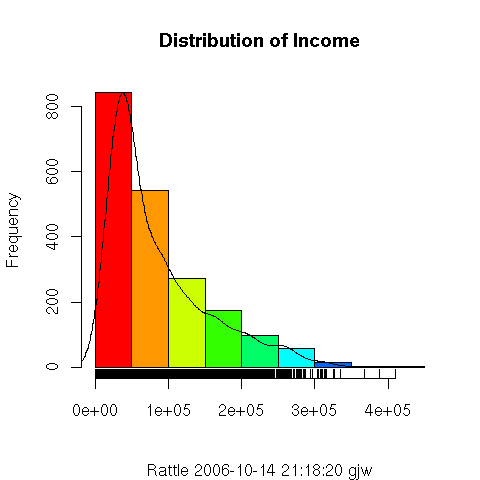A histogram provides a quick and useful graphical view of the spread of the data. A histogram plot in Rattle includes three components. The first of these is obviously the coloured vertical bars. The continuous data in the example here (Distribution of Income) has been partitioned into ranges, and the frequency of each range is displayed as the bar. R is automatically choosing both the partitioning and how the x-axis is labelled here, showing x-axis points at 0, 10,000 (using scientific notation of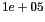which means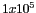, or 10,000), and so on. Thus, we can see that the most frequent range of values is in the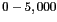partition. However, each partition spans quite a large range (a range of \$5,000).

The plot also includes a line plot showing the so called density estimate and is a more accurate display of the actual (at least estimated true) distribution of the data (the values of Income). It allows us to see that rather than values in the rangeoccurring frequently, in fact there is a much smaller range (perhaps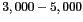) that occurs very frequently.

The third element of the plot is the so called rug along the bottom of the plot. The rug is a single dimension plot of the data along the number line. It is useful in seeing exactly where data points actually lay. For large collections of data with a relatively even spread of values the rug ends up being quite black, as is the case here, up to about \$25,000. Above about \$35,000 we can see that there is only a splattering of entities with such values. In fact, from the Summary option, using the Describe check box, we can see that the highest values are actually \$36,1092.60, \$38,0018.10, \$39,1436.70, \$40,4420.70, and \$42,1362.70.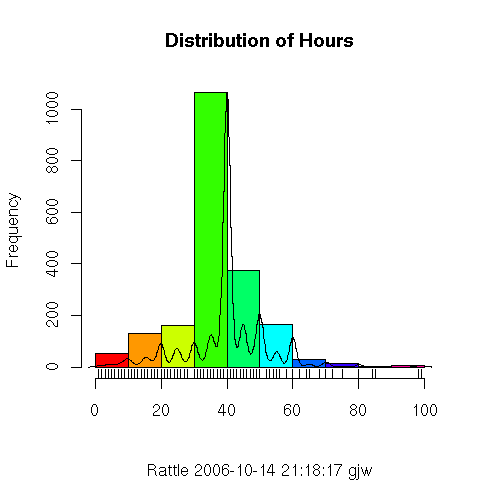This second plot, showing the distribution for the variable Hours, illustrates a more normal distribution. It is, roughly speaking, a distribution with a peak in the middle and diminishing on both sides, with regards the frequency. The density plot shows that it is not a very strong normal distribution, and the rug plot indicates that the data take on very distinct values (i.e., one would suggest that they are integer values, as is confirmed through viewing the textual summaries in the Summary option).